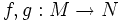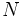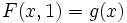# Smooth homotopy

Suppose$M,N$ are differential manifolds, and$f,g: M \to N$ are smooth maps. A smoooth homotopy from$f$ to$g$ is a smooth map from$M \times I$ (viewed with the product manifold structure) to$N$ such that$F(x,0) = f(x)$ and$F(x,1) = g(x)$ for all$x$.
In other words, a smooth homotopy is a homotopy from$f$ to$g$ (in the topological sense) which is also a smooth map when viewed with the additional structure of a manifold.